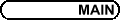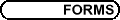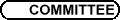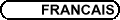Approximation Theory / Théorie d'approximation
Org: Richard Fournier and/et Paul Gauthier (Montreal)

THOMAS BLOOM, University of Toronto, 100 St. George Street, Toronto, ON M1M 3W5
Random Polynomials and Green Functions

Let K be a regular (in the sense of pluripotential theory) compact set in Cn and let VK(z) denote its pluricomplex Green function with a logarithemic singularity at ¥. Then, with probability 1, a sequence of random polynomials {fa} gives the pluricomplex Green function via the formula

 æè \varlimsupa 1 deg(fa) log|fa(z)| öø * = VK (z)     for all z Î Cn
In the one-dimensional case, this result may be used to generalize a result of Shiffman-Zelditch on the limiting normalized distributon of zeroes of random polynomials.

ANDRÉ BOIVIN, Univerity of Western Ontario
Approximation on Riemann surfaces: recent results

Some recent results concerning approximation on Riemann surfaces will be presented. These will include generalizations to Riemann surfaces of a theorem of A. G. Vitushkin on uniform approximation by rational (meromorphic) functions, and of results by T. W. Gamelin and J. B. Garnett on bounded pointwise approximation.

This is joint work with Jiang B.

MAXIM BURKE, University of Prince Edward Island, Charlottetown, PE C1A 4P3
Entire functions mapping uncountable dense sets of reals onto each other monotonically

When A and B are countable dense subsets of R, it is a well-known result of Cantor that A and B are order-isomorphic. A theorem of K. F. Barth and W. J. Schneider states that the order-isomorphism can be taken to be very smooth, in fact the restriction to R of an entire function. J. E. Baumgartner showed that consistently 2À0 > À1 and any two subsets of R having À1 points in every interval are order-isomorphic. However, U. Abraham, M. Rubin and S. Shelah produced a ZFC example of two such sets for which the order-isomorphism cannot be taken to be smooth. A useful variant of Baumgartner's result for second category sets was established by S. Shelah. He showed that it is consistent that 2À0 > À1 and second category sets of cardinality À1 exist while any two sets of cardinality À1 which have second category intersection with every interval are order-isomorphic. In this paper, we show that the order-isomorphism in Shelah's theorem can be taken to be the restriction to R of an entire function. Moreover, using an approximation theorem of L. Hoischen, we show that given a nonnegative integer n, a nondecreasing surjection g :R ® R of class Cn and a positive continuous function e: R ® R, we may choose the order-isomorphism f so that for all i = 0,1,...,n and for all x Î R, |Di f(x)-Di g(x)| < e(x).

DIMITER DRYANOV, Concordia University, 7141 Sherbrooke Street West, Montreal, QC H4B 1R6
A Refinement of an Inequality of R. J. Duffin and A. C. Schaeffer

Let p be a polynomial of degree n. Let ms : = n-1 sin-2( sp/(2n) ), s odd, 1 £ s < n, and mn = (1-(-1)n ) / (4n). By using ms and xj = cos(jp/n), j integer, we define the linear functionals

 Lj (p) = nå s=1, s odd ms æè p(xj) - p(xj+s) + p(xj-s) 2 öø
for j = 0,1,...,n.

The following inequality is established:

 max x Î [-1,1] |p¢(x) | £ n max 0 £ j £ n |Lj(p) |.

Remark 1 In view of

 nå s=1, s odd ms = n 2
and by making use of the sets
 To : = {xj : j odd, 0 £ j £ n},     Te : = {xj : j even, 0 £ j £ n}
we have:
 max x Î [-1,1] | p¢(x) |
 £ n max 0 £ j £ n | Lj(p) |
(1)
 £ n2 2 max x Î Co, y Î Ce |p(x)-p(y)| £ n2 max 0 £ j £ n |p(xj)|.
(2)

Remark 2 The inequality obtained is a refinement of the well known inequality:

 max x Î [-1,1] |p¢(x) | £ n2 max 0 £ j £ n |p(xj)|
which is due to Duffin and Schaeffer .

## References


R. J. Duffin and A. C. Schaeffer, A refinement of an inequality of the brothers Markoff. Trans. Amer. Math. Soc. 50(1941), 517-528.

SERGE DUBUC, Université de Montréal, C.P. 6128, Succ. Centre-ville, Montréal, Québec H3C 3J7
The joint spectral radius of a family of matrices

Let A,B be the two 2×2 matrices

æ
ç
è
 1+2l
 2l
 -1/2-2l+m/2
 1/2-2l-m/2
ö
÷
ø
,     æ
ç
è
 1/2-2l-m/2
 -1/2-2l+m/2
 2l
 1+2l
ö
÷
ø
,
we define the subset of the plane W = {(l,m) :r(A,B) < 1} where r(A,B) is the joint spectral radius of {A,B}. We discuss the approximation of W.

RICHARD FOURNIER, CRM, Université de Montréal, C.P. 6128, Succ.  Centre-ville, Montréal, Québec H3C 3J7
A New Inequality for Polynomials

Let D be the unit disc of the complex plane C. We prove that for any polynomial p of degree at most n

 max q Î R êê p(eiq) - p(e-iq) eiq - e-iq êê £ n max 0 £ j £ n êê p(eijp/n) + p(e-ijp/n) 2 êê .
We shall also discuss how this inequality is related to classical results or Bernstein and Markov and to more recent ones due to Duffin and Schaeffer, Frappier, Rahman and Ruscheweyh.

This is joint work with Dimiter Dryanov.

PAUL GAUTHIER, Université de Montréal, Centreville, Montréal, QC H3C 3J7
Approximation of and by the Riemann zeta function

Firstly, we approximate the Riemann zeta function by meromorphic functions for which the Riemann hypothesis fails. Secondly, we approximate arbitrary holomorphic functions by linear combinations of translates of the Riemann zeta function. The first result is joint work with E. S. Zeron. The second work is joint with N. N. Tarkhanov.

DAN KUCEROVSKY, University of New Brunswick
Quasi-monotone sequences

Functions from finite sets to Rm occur very frequently in applied problems. If m=1, then there is a standard definition of monotonicity, and it is often useful to break up the function into (approximately) monotone segments. For the case of higher dimensional range spaces, there is no order structure, so we instead consider breaking up the functions into segments of bounded curvature. This leads to the problem of determining a bound on the curvature of a segment in a computationally efficient way, which we do by a recursive formula. We compare with quasi-linear fitting, obtained by a least-squares method, and find that the curvature method is more robust, in particular, with respect to deletion of data points.

Joint work with Daniel Lemire, UQAM.

Zeros of functions in the Dirichlet space

There are several uniqueness theorems for functions in the classical Dirichlet space. But, a necessary and sufficient condition (like the Blaschke condition for Hardy spaces) for this space is not yet available. We will discuss a new uniqueness theorem.

This is a joint work with Thomas Ransford and Abdellatif Bourhim.

THOMAS RANSFORD, Université Laval, Dép. de mathématiques, Québec (QC), G1K 7P4
A Denjoy-Carleman maximum principle

We prove a quantitative form of the classical Denjoy-Carleman theorem on quasi-analytic classes. As an application, we derive an extension of Carleman's theorem on the unique determination of probability measures by their moments.

Joint work with Isabelle Chalendar, Laurent Habsieger and Jonathan Partington.

GERALD SCHMIEDER, Universitaet Oldenburg, Fak. V, Inst. f. Math., 26111 Oldenburg, Germany
Extension of the Fusion Lemma

Let K1, K2 be compact sets in the complex plane C. We say that K1, K2 is a fusion pair if there exists some constant a = a(K1,K2) with the following property: for all rational functions r1, r2 and for each compact set K Ì C there is a rational function r which fulfills ||rj-r||KjÈK £ a·||r1-r2||K simultaneously for j=1,2.

Alice Roth proved in 1976 that K1,K2 is a fusion pair if the sets K1, K2 are disjoint. If K1ÇK2 = Æ is not required we have of course to replace ||r1-r2||K above by ||r1-r2||KÈ(K1ÇK2). But in general K1, K2 is no fusion pair (examples are due to Gauthier and Gaier).

Under rather natural topological restrictions (especially that K1,K2 have no common interior points) we can characterize the fusion pairs by the simple condition that K1 ÇK2 = (K1ÈK2).

JIE XIAO, Memorial University of Newfoundland, St. John's, NL A1C 5S7
The Heat Equation II: Regularity and Approximation

We use the Carleson-Sobolev estimates for an operator valued solution of the heat equation to give the regularity of the solution and its application to the Weierstrass approximation theorem.

EDUARDO ZERON, Cinvestav (Math), Apartado postal 14-740, Mexico DF, 07000, Mexico
Homotopical obstructions to rational approximation

There are several criteria to decide whether a continuous function F(z) defined from a compact set K Ì Cn into C can be approximated by holomorphic rational functions (P/Q)(z). Now, any rational function (P/Q)(z) can be seen as a holomorphic function defined from an open set of Cn into the Riemann sphere S2. And we can even generalise the concept of rational function to consider holomorphic functions with range into the complex projective space CPn.

We may then ask about the rational approximation of continuous functions G(z) defined from a compact set K Ì Cn into CPn. We want to show, in this talk, that there is essentially one extra necessary condition on G(z) to be approximated by rational functions: Function G must be homotopically trivial.

PING ZHOU, St. Francis Xavier University, Antigonish, NS B2G 2W5
Divided differences in construction of multivariate Padé approximants

We explicitly construct multivariate Padé approximants to some functions of the form

 F(x1,x2,...,xm) = ¥å n=0 f(n) å j1+j2+¼ +jm=n x1j1 x2j2 ¼xmjm,
where f(n) is a certain function of n, by using divided differences. Examples include the multivariate exponential function
 E(x1,x2,...,xm) : = ¥å j1,j2,...,jm=0 x1j1 x2j2 ¼xmjm (j1+j2+¼ +jm+1)! ,
the multivariate logarithm function
 L(x1,x2,...,xm) : = ¥å j1,j2,...,jm=0 x1j1 x2j2 ¼xmjm j1+j2+¼+jm+1 ,
and others.